Next: Dirac Equation Up: Relativistic Electron Theory Previous: Introduction

# Preliminary Analysis

In the following, we shall employ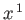,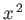,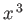to represent the Cartesian coordinates,,, respectively, and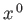to represent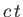, where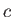is the velocity of light in vacuum. The time-dependent wavefunction then takes the form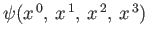. Adopting standard relativistic notation, we write the four's as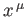, for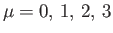. A space-time vector with four components that transform under Lorentz transformation in an analogous manner to the four space-time coordinates,, is termed a 4-vector , and its components are written like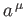(i.e., with an upper Greek suffix). We can lower the suffix according to the rules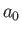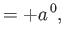(11.1)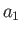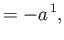(11.2)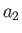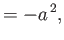(11.3)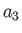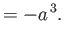(11.4)

Here, theare called the contravariant components of the 4-vector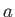, whereas theare termed the covariant components. Two 4-vectorsand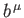have the Lorentz-invariant scalar product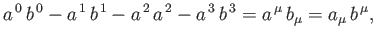(11.5)

a summation being implied over a repeated letter suffix . The metric tenor,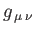, is defined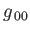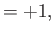(11.6)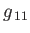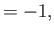(11.7)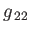(11.8)(11.9)

with all other components zero . Thus,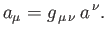(11.10)

Likewise,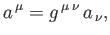(11.11)

where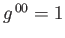,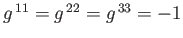, with all other components zero. Finally,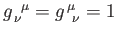if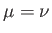, and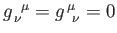otherwise.

In the Schrödinger representation (see Section 2.4), the momentum of a particle, whose Cartesian components are written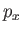,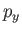,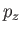, or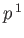,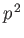,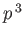, is represented by the operators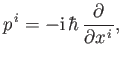(11.12)

for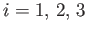. Now, the four operators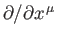form the covariant components of a 4-vector whose contravariant components are written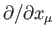. (See Exercise 1.) So, to make expression (11.12) consistent with relativistic theory, we must first write it with its suffixes balanced,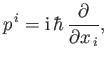(11.13)

and then extend it to the complete 4-vector equation,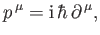(11.14)

where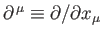. According to standard relativistic theory, the new operator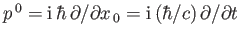, which forms a 4-vector when combined with the momenta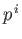, is interpreted as the energy of the particle divided by.Next: Dirac Equation Up: Relativistic Electron Theory Previous: Introduction
Richard Fitzpatrick 2016-01-22